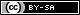# Dev Blog

 ./dev Original theme by orderedlist (CC-BY-SA) Where applicable, all content is licensed under a CC-BY-SA.# Kullback-Leibler Divergence

Here is a short discussion of the Kullback-Leibler divergence that helps give some motivation for what it is an where it comes from.

Given two (discrete) probability distribution functions, $p(\cdot)$ and $q(\cdot)$, the Kullback-Leibler divergence is defined as:

$$D_{KL}(p || q) = - \sum_{x} p(x) \ln(\frac{p(x)}{q(x)})$$

The Kullback-Leibler divergence, in some intuitive sense, measures the difference between probability distributions.

As motivation, consider a situation where you are providing internet service to a business. This business wants to compress their data but has, incorrectly, guessed at a distribution $q(x)$ for the symbols it's trying to transmit.

If you've determined the true distribution to be $p(x)$ the question is, how much is the gain you get by, say, charging the business for compressing their data with the probability distribution $q(x)$ when you have knowledge and can use the true distribution $p(x)$.

The business will encode it's messages with an average of

$$-\sum_{x} p(x) \lg(q(x))$$

bits, where the $\lg(q(x))$ is the encoded bit size of the token $x$ but will only happen with the true underlying distribution $p(x)$. If you take the messages and re-encode them properly, the average bit size will be:

$$-\sum_{x} p(x) \lg(p(x))$$

Taking the difference:

$$\begin{array}{cl} & -\sum_{x} p(x) \lg(p(x)) - [-\sum_{x} p(x) \lg(q(x) ] \\ \to & -\sum_{x} p(x) \lg( \frac{p(x)}{q(x)}) \end{array}$$

On average, $D_{KL}(p || q)$ bits per token are saved. If the business is willing to pay rates based on the bits they see going out, namely $-\sum_{x} p(x) \lg(q(x))$, but you're paying only for $-\sum_{x} p(x) \lg(p(x))$ bits going out, then there's potential profit on the difference to be made.

The cross entropy is a concept closely connected to the Kullback-Leibler distribution.

The cross entropy is defined as:

$$H(p,q) = -\sum_{x} p(x) \lg(q(x))$$

The Kullback-Leibler distribution can be seen as the difference of the entropy of the distribution $p(\cdot)$ and the cross entropy of $p(\cdot)$ and $q(\cdot)$:

$$\begin{array}{cl} D_{KL} & = H(p) - H(p,q) \\ & = -\sum_{x} p(x) \lg(\frac{p(x)}{q(x)}) \end{array}$$

In the above example, the cross entropy is how many bits the business is transmitting under their fallacious assumption of $q(\cdot)$ as the distribution and the entropy of $p(\cdot)$ is the "optimized" bits you transmit with knowledge of the underlying distribution.

When given a "guess" distribution, $q(x)$, one can compare how "bad" it is compared with a "true" or underlying distribution, $p(x)$.